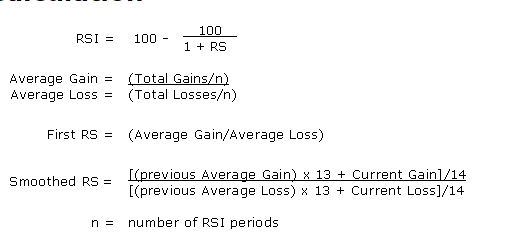# Calculation for RSI,ADX and PSAR

How to calculate RSI,ADX and PSAR using the history data.
Tagged:
• 1. get tick data from market
2. parse the data
3. convert it into ohlc of the timeframe you need
4. use this formula for rsi ...similarly calculate for adx and pasr
• How to calculate ADX and PASR?